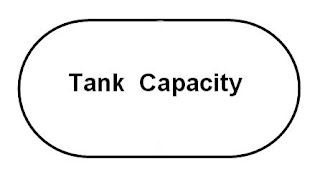## Kamis, 23 Mei 2013

### Calculate of volume horizontal cylindrical tank with hemispherical end caps

Calculate of volume horizontal cylindrical tank with hemispherical end caps.

The following picture above is an hemispherical  tank type  is mounted horizontally,
Tank with an hemispherical   type cross-section of different ways to calculate than
with a cylindrical tank.

Advantage  of this type of tank, better than the tank type of box, fluid can be drained
away due design the bottom  tank is smaller.

how to find the liquid volume calculation, description of the calculation is the same as
silinder tank horizontal, just need to add a piece of the ball at the end .

Here's what formula is used, after the ball ( hemispherical end caps ) on the plus
section ends :

As noted in the previous post, the following i am provide applications to calculate
the volume of liquid in silinder hemispherical  tank the Horizontal. As in the
following  screenshoot:

http://www.4shared.com/rar/VL8-ApJa/Tanki_hemisperical.html

The following graphs below, display the liquid level rise compared with
the volume of liquid.  With data input Length of cilindre (L) = 200 cm,
radius cilindre ( R )= 50 cm, radius of end caps ( R2 ) = 30 cm,
height of  liquid level ( h ) = variable data.

As per screenshoot below, we make compare results application calculation
based on same dimension input data. based input data , liquid level = 30 cm.

Thanks for visit my blog